# How to split my value from two types of string?

I have to get name and surname informations from my string. I have two types of string format.
First one includes “1111 Name Surname” and the other one includes “1111 Name1 Name2 Surname”
I should get all names and surnames. How to get second one?

Hello @ozgecatak

Can you explain with a real example?

Here if it separated with space then you can use the Split command.

myVal=Split(“Yourstring”," ");

myVal is the string array.

Then use the index to get the value. myVal(0),myVal(1) etc.

Thanks

The following are my real examples

Example 1: my_str1= “9919 Ozge Ctk”

Example 2: my_str2= “9919 Mert Alp Ctk”

I know first one but I dont know second one.

You can try with Regex expression

Use Assign activity

``````StrVal = System.Text.RegularExpressions.Regex.Match(my_str2,"\s\D+").Tostring.trim
``````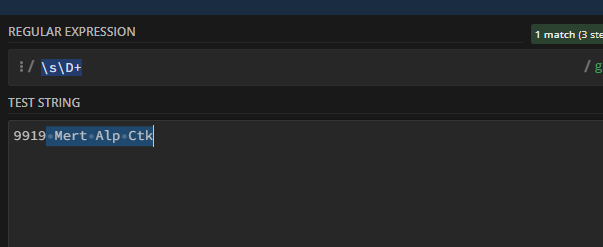Regards
Gokul

Hello @ozgecatak

Here you can use regex to get the required value or you can split with space and then concatenate.

``````system.Text.RegularExpressions.Regex.Match(Yourstring, "\s\D+").Value;
``````

Thanks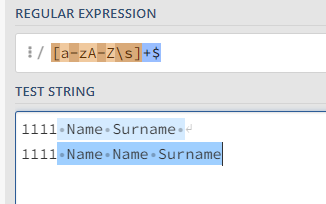Use regex

``````TotalName= System.Text.RegularExpressions.Regex.Match(YourString,"\D+").ToString.Trim
``````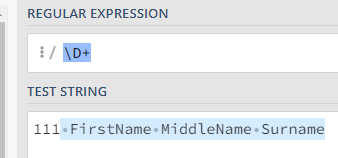For last name or surname
`LastName= Split(TotalName.Trim," ").Last`
For First name and middle name
`FirstName= TotalName.Replace(LastName.Trim,"").ToString.Trim`

1 Like

Thanks. How about my value was like my_str= “9919 Ozge Eda Ctk ( XXX yyy Zzzz)”
And how to get Ozge Eda Ctk. But dont forget, Ozge Eda Ctk is not fixed word. It is changeable.

@ozgecatak
Try this

\D+(?=()

``````TotalName= System.Text.RegularExpressions.Regex.Match(my_str,"\D+(?=\()").ToString.Trim
``````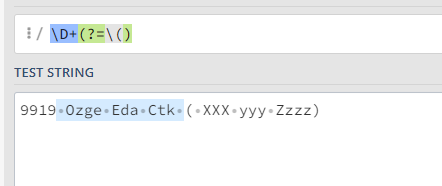For the above two cases

``````TotalName= System.Text.RegularExpressions.Regex.Match(my_str,"(?<=\d\s).*(?=\()|(?<=\d\s).*").ToString.Trim
``````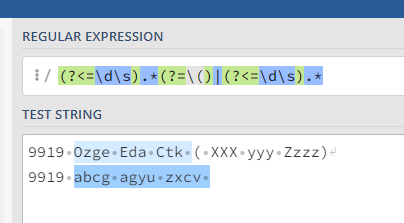1 Like

``````TotalName= System.Text.RegularExpressions.Regex.Match(my_str,"(?<=\d{4}\s).*(?=\s\()|(?<=\d{4}\s).*").ToString.Trim
``````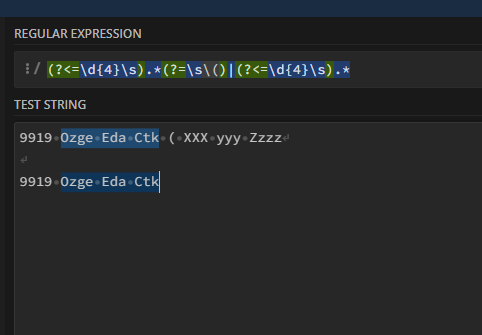1 Like

I use it thank you

Do you have any other query?

If not, Kindly close this topic by marking solved @ozgecatak

Regards
Gokul

This topic was automatically closed 3 days after the last reply. New replies are no longer allowed.